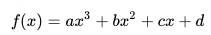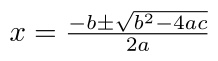# Transcendental Functions & Algebraic Functions: Simple Definition

Functions can be separated into two types: algebraic functions and transcendental functions.

## What is an Algebraic Function?

Algebraic functions are built from finite combinations of the basic algebraic operations: addition, subtraction, multiplication, division, and raising to constant powers.

Three important types of algebraic functions:

• Polynomial functions, which are made up of monomials. The terms can be made up from constants or variables. For example, 2x + 1, xyz + 50, f(x) = ax2 + bx + c . Every subtype of polynomial functions are also algebraic functions, including:
• Linear functions, which create lines and have the form y = mx + b,
• Cubic functions, third degree polynomials which have the form• Quadratic functions: fourth degree polynomials with the form.
• Power functions: any function of the form f(x) = axp, where: a and p are constants, p is a real number,
and a is nonzero.
• Rational functions: any function where one polynomial function is divided by another.

Unfortunately, the incomplete rules of integration in calculus are not sufficient for integrating simple algebraic functions like √(1 + x3). This shouldn’t come as a surprise seeing that the rules also fail at finding other basic functions, like rational functions with undetermined constants (e.g. 1/(x5 – x – A). It is only relatively recently (in the last few decades) that mathematicians have been able to identify which algebraic functions can be integrated by the usual rules. Even then, the forms which these solutions take are not suitable for calculus textbooks .

## What is a Transcendental Function?Most mathematicians would agree that the functions on this list are examples of transcendental functions, only because they aren’t algebraic.The exact definition of what a transcendental function is is hard to pin down. That’s because it’s generally defined by exclusion: If a function isn’t algebraic, then it’s transcendental.

I say “generally”, because there isn’t an exact consensus, and individual authors may state a certain function is transcendental, while another may disagree. Some authors might state that transcendental functions are generated by infinite series; While that is true, it’s also true that some algebraic functions can be generated by infinite series as well. Stillwell  states that the most important transcendental functions (which he lists as exponential functions, logarithmic functions, circular functions and hyperbolic functions) are obtained by integrating and inverting “fairly simple” algebraic functions. For example, ey is the inverse function of y = log x, andHowever, not all transcendental functions can be obtained in this manner, which means the definition of “integrate and invert” isn’t complete either.

While algebraic functions are a set of small, precisely defined functions (e.g. constant functions, exponential functions), transcendentals are simply “everything else.”

According to a 2001 post by Dr. Math, there are also several “named” transcendental functions. For example: Bessel functions and Hankel functions. These are usually only found in very specialized areas, so if you’re in an elementary calculus class, you’re unlikely to come across them.

A few examples of functions that most people agree are transcendental in nature include:

## Why are they called Transcendental Functions?

Transcendental, in math, means “non-algebraic”. These functions “transcend” the usual rules of algebra (transcend means to “go beyond the range or limits of…”). Transcendentals were first defined by Euler in his Introductio (1748) as functions not definable by the “ordinary operations of algebra”.

## Limits of Transcendental Functions

Limits of Transcendental Functions can be found with direct substitution.

## References

 Stillwell, J. Mathematics and Its History. 3rd Edition. Springer.

Dr. Math (2001). Defining Transcendental Functions. Retrieved May 22, 2019 from: http://mathforum.org/library/drmath/view/54593.html
Larson, R. & Edwards, B. Calculus: Early Transcendental Functions. Cengage Learning. Retrieved May 22, 2019 from: https://books.google.com/books?id=zUfAAgAAQBAJ

CITE THIS AS:
Stephanie Glen. "Transcendental Functions & Algebraic Functions: Simple Definition" From StatisticsHowTo.com: Elementary Statistics for the rest of us! https://www.statisticshowto.com/transcendental-functions/